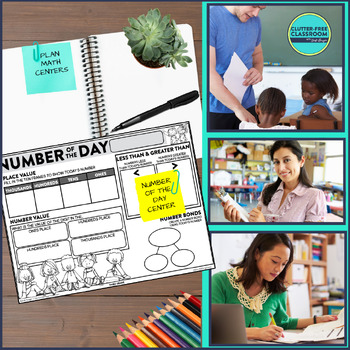# Number of the Day Google Slides and Worksheets for 3rd Grade3rd, Homeschool
Subjects
Standards
Resource Type
Formats Included
• Zip
• Internet Activities
•Google Apps™
Pages
10 pagesThe Teacher-Author indicated this resource includes assets from Google Workspace (e.g. docs, slides, etc.).

#### Also included in

1. This 3rd grade math bundle includes math assessments, journals, spiral review problems, vocabulary activities and more. It's a huge collection of printable and digital math activities to use with your third graders! With this resource you'll have a go to library of resources to save you tons of time
\$79.80
\$116.99
Save \$37.19

### Description

Using these 3rd grade number of the day templates, which are available as Google Slides and printable worksheets in this resource, supports third grade students in developing a strong number sense and understanding of the most important grade level math concepts and skills. The best part is that the number of the day Google Slides follow the same template as the number of the day printable activities and can be assigned for remote learning, so your students can access them with ease and understand the expectations even if their learning situation changes.

Use these third grade number of the day activities for

◼️ whole group lessons

◼️ do now activities

◼️ homework assignments

◼️ math center work

◼️ independent practice

◼️ remote learning activities

WHAT IS INCLUDED:

✔ 5 Digital pages that can be used for in-person or remote learning. It serves as a great whole group in-class lesson on your interactive white board or projected on your non-tech white board.

✔ 5 Printable pages that are completely print and go and can be used for morning work, math centers, homework and more

✔ Open-ended questions that make it easy to differentiate the activities to meet the needs of your students by changing the size and complexity of the number and make it so you can use the activities all year long

5 REASONS TEACHERS LOVE THIS RESOURCE:

► The printable activities require no prep - Just print and go! This will save you lots of time!

► The activities create opportunities for students to practice some of the most important skills for their grade level.

► They can be used for remote and in-person learning, so they can be used no matter what your teaching situation looks like.

► They can be used as morning work, homework, independent practice, remote learning and more.

◼️THE EMAIL CLUB → weekly freebies

◼️FACEBOOK GROUP → a supportive community of elementary teachers

◼️THE TPT STORE → new resources 50% off

◼️THE BLOG → teaching tips and ideas

◼️INSTAGRAM → a look behind the scenes

Total Pages
10 pages
N/A
Teaching Duration
1 Year
Report this Resource to TpT
Reported resources will be reviewed by our team. Report this resource to let us know if this resource violates TpT’s content guidelines.

### Standards

to see state-specific standards (only available in the US).
Fluently multiply and divide within 100, using strategies such as the relationship between multiplication and division (e.g., knowing that 8 × 5 = 40, one knows 40 ÷ 5 = 8) or properties of operations. By the end of Grade 3, know from memory all products of two one-digit numbers.
Understand division as an unknown-factor problem. For example, find 32 ÷ 8 by finding the number that makes 32 when multiplied by 8.
Apply properties of operations as strategies to multiply and divide. Examples: If 6 × 4 = 24 is known, then 4 × 6 = 24 is also known. (Commutative property of multiplication.) 3 × 5 × 2 can be found by 3 × 5 = 15, then 15 × 2 = 30, or by 5 × 2 = 10, then 3 × 10 = 30. (Associative property of multiplication.) Knowing that 8 × 5 = 40 and 8 × 2 = 16, one can find 8 × 7 as 8 × (5 + 2) = (8 × 5) + (8 × 2) = 40 + 16 = 56. (Distributive property.)
Determine the unknown whole number in a multiplication or division equation relating three whole numbers. For example, determine the unknown number that makes the equation true in each of the equations 8 × ? = 48, 5 = __ ÷ 3, 6 × 6 = ?.
Use multiplication and division within 100 to solve word problems in situations involving equal groups, arrays, and measurement quantities, e.g., by using drawings and equations with a symbol for the unknown number to represent the problem.